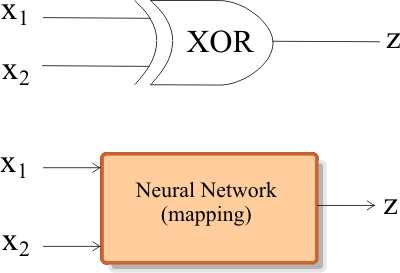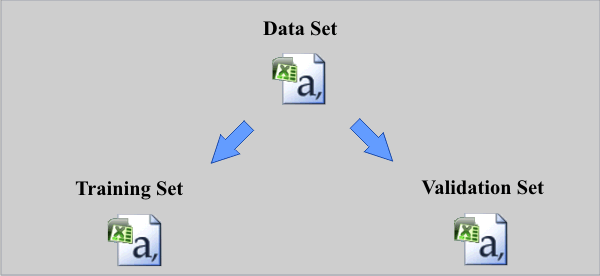# Logic Operation XOR

## XOR Gate

In its most basic configuration, the gate has two inputs and one output. The output is one when one input is different from the other input as shown below.x1 x2 z 0 0 0 0 1 1 1 0 1 1 1 0

 Problem 1 Create a New Project called MappingXor to build an appropriate set for the XOR gate. Use 256 cases. Add the BuildDataSet.lab file to your project and run the program.

 Problem 2 Add a new code file called Split.lab to build the training set and the validation set from the data set. Use 75 % of the cases for training, and 25 % of the cases for validation, see figure below.Problem 3 Edit the Train.lab file to design and train an ANN for the XOR gate. Use one hidden layer and one neuron in this layer. Train the ANN using simulated annealing as shown:      Number of iterations = 100      Number of Temperatures = 100      Initial Temperature = 15      Final Temperature = 0.01      Cooling Schedule = Linear      Number of Cycles = 4      Goal (desired mse) = 0.00001

 Problem 4 Edit the CheckTraining.lab file to check the training: (a) Compute the mean squared error for the ANN using the training set. (b) Plot the error for each training case. (c) Save the plot as a vector image (checkTraining.pdf and checkTraining.emf)

 Problem 5 Edit the Validation.lab file to perform the validation of the ANN. (a) Compute the mean squared error for the ANN using the validation set. (b) Plot the error for each validation case. (c) Save the plot as a vector image (validation.pdf and validation.emf)

 Problem 6 Repeat problem 3, 4 and 5 using two neurons in the hidden layer. What can be concluded from the results of the last problems?

 Problem 7 Generate a report in Microsoft Word. Write some conclusions in the report focusing on the problems that were faced during the simulation and how these problems were or could be solved.